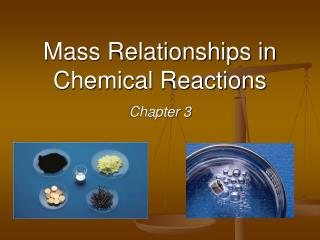# Mass Relationships in Chemical Reactions - PowerPoint PPT PresentationDownload PresentationMass Relationships in Chemical Reactions

Download Presentation## Mass Relationships in Chemical Reactions

- - - - - - - - - - - - - - - - - - - - - - - - - - - E N D - - - - - - - - - - - - - - - - - - - - - - - - - - -
##### Presentation Transcript

1. Formula Weight (FW) • Sum of the atomic weights for the atoms in a chemical formula. • e.g., formula weight of calcium chloride, CaCl2 Ca: 1(40.1 amu) + 2Cl: 2(35.5 amu) 111.1 amu • Formula weights used for ionic or molecular compounds

2. + 6H: 6(1.0 amu) Molecular Weight (MW) • Sum of the atomic weights of the atoms in a molecule. • For the molecule ethane, C2H6, the molecular weight would be C: 2(12.0 amu) 30.0 amu • Formula weights used for molecular compounds only

3. (number of atoms)(atomic weight) x 100% % element = (FW of the compound) Percent Composition Percentage mass of a compound that comes from each of the elements in the compound:

4. (2)(12.0 amu) %C = (30.0 amu) x 100% 24.0 amu = 30.0 amu Percent Composition For the molecule ethane, C2H6, with MW = 30.0 amu = 80.0% C in ethane

5. Dozen = 12 Pair = 2 Micro World (atoms & molecules) Macro World (grams) The mole (mol) - amount of a substance that contains as many particles as there are atoms in exactly 12.00 grams of 12C 1 mol = 6.02 x 1023 Avogadro’s number (NA)

6. Avogadro’s Number • 1 carbon atom has a mass of 12.0 amu • 1 mole of 12C has a mass of 12.0 g Micro World (atoms & molecules) Macro World (grams)

7. Using Moles • Moles provide a bridge from the molecular scale to the real-world scale.

8. How many moles of calcium (Ca) are in 5.0750 g of calcium? (Z = 20) From periodic table: 1 mol Ca = 40.078 g Ca

9. atoms ions Molar mass - the mass of 1 mole of in grams molecules formula units 1 mole 12C atoms = 6.022 x 1023 atoms = 12.00 g 1 mole S atoms = 32.066 g S 1 mole lithium atoms = 6.941 g of Li For any element: atomic mass (amu) = molar mass (grams)

10. (Average) atomic mass (6.941)

11. Do You Understand Molar Mass? 1 mol K x 39.0983 g K How many moles are in 0.551 g of potassium (K) ? 1 mol K = 39.0983 g K 0.551 g K = 1.41 x 10-2 mol K

12. 1S 32.07 amu 2O + 2 (16.00 amu) SO2 SO2 64.07 amu Molecular mass (or molecular weight) - the sum of the atomic masses (in amu) in a molecule. For any molecule: molecular mass (amu) = molar mass (grams) 1 molecule SO2 = 64.07 amu 1 mole SO2 = 64.07 g SO2

13. Do You Understand Molar Mass? 1 mol C3H8O 60.0 g C3H8O How many moles are in 72.5 g of C3H8O ? 1 mol C3H8O = (3 mol C) (12.0 g/mol) + (8 mol H) (1.0 g/mol) + (1 mol O) (16.0 g/mol) 1 mol C3H8O = 60.0 g C3H8O = 1.21 mol C3H8O (72.5 g C3H8O)

14. Do You Understand Molar Mass? What is the mass of 0.433 mol of Ca(NO3)2 ? 1 mol Ca(NO3)2 = (1 mol Ca) (40.078 g/mol) + (2 mol N) (14.01 g/mol) + (6 mol O) (16.00 g/mol) = 164.1 g Ca(NO3)2 164.1 g Ca(NO3)2 0.433 mol Ca(NO3)2· = 71.1 g Ca(NO3)2 1 mol Ca(NO3)2